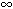DOC HOME SITE MAP MAN PAGES GNU INFO SEARCH PRINT BOOK

# floor(3M)

floor, ceil, copysign, fmod, fabs, nan, nearbyint, nextafter, nexttoward -- return various results from calculations on arguments

## Synopsis

```   cc [flag ...] file ...  -lm [library ...]

#include <math.h>

double floor(double x);

float floorf(float x);

long double floorl(long double x);

double ceil(double x);

float ceilf(float x);

long double ceill(long double x);

double copysign(double x, double y);

float copysignf(float x, float y);

long double copysignl(long double x, long double y);

double fmod(double x, double y);

float fmodf(float x, float y);

long double fmodl(long double x, long double y);

double fabs(double x);

float fabsf(float x);

long double fabsl(long double x);

double nan(const char *tagp);

float nanf(const char *tagp);

long double nanl(const char *tagp);

double nearbyint(double x);

float nearbyintf(float x);

long double nearbyintl(long double x);

double nextafter(double x, double y);

float nextafterf(float x, float y);

long double nextafterl(long double x, long double y);

double nexttoward(double x, long double y);

float nexttowardf(float x, long double y);

long double nexttowardl(long double x, long double y);
```

## Description

floor, floorf and floorl return the largest integer not greater than x. ceil, ceilf and ceilf return the smallest integer not less than x.

copysign, copysignf and copysignl return x but with the sign of y.

fmod, fmodf and fmodl return the floating point remainder of the division of x by y. More precisely, they return the number f with the same sign as x, such that x = iy + f for some integer i, and |f| < |y|.

fabs, fabsf and fabsl return the absolute value of x, |x|.

nan, nanf and nanl return a quiet NaN, if available, with content indicated by tagp. Using a call such as nan("n-char-sequence") is equivalent to strtod("NAN(n-char-sequence)", (char**) NULL). Using a call such as nan("") is equivalent to strtod("NAN()", (char**) NULL). If tagp does not point to an n-char sequence or an empty string, the call is equivalent to strtod("NAN", (char**) NULL).

The same applies to nanl that behaves the same as strtold.

nearbyint, nearbyintf and nearbyintl return the nearest integer value to its floating point argument x as a double-precision, single-precision or long double-precision number. The returned value is rounded according to the currently set machine rounding mode. If round-to-nearest (the default mode) is set and the difference between the function argument and the rounded result is exactly 0.5, then the result will be rounded to the nearest even integer.

nextafter, nextafterf and nextafterl determine the next representable value, in the type of the function, after x in the direction of y, where x and y are first converted to the type of the function. These functions return y if x equals y. A range error can occur if the magnitude of x is the largest finite value representable in the type and the result is infinite or not representable in the type.

nexttoward, nexttowardf and nexttowardl are equivalent to nextafter, nextafterf and nextafterl except that the second parameter (y) is a long double. If x equals y then y is first converted to the type of the function before being returned.

### Errors

When x is ±or y is zero fmod, fmodf and fmodl return IEEE NaN on systems that support it and raise the invalid operation exception. Otherwise, they return 0. errno is set to EDOM.

On systems that support IEEE NaN, if any of the inputs to each of these functions is a quiet NaN, that value is returned. If any of the inputs is a signaling NaN, a quiet NaN is returned and the invalid operation exception is raised. In either case, errno is set to EDOM.

If the program was compiled with the -Xt compilation mode, fmod, fmodf and fmodl return x when y is zero and set errno to EDOM. In addition, a message indicating DOMAIN error is printed on the standard error output. These error handling procedures may be changed with the function matherr.

## References

abs(3C), cc(1), matherr(3M), strtod(3C), strtold(3C)